MAA Minicourse #7
¤
January 7 and 9, 2016
Making Sense of Calculus with Mapping Diagrams
Part II
Integration, Series, and Multi-Variable Calculus

Martin Flashman
Professor of Mathematics
Humboldt State University

Abstract:
In this mini-course participants will learn how to use mapping diagrams (MD) to visualize functions for many calculus concepts. For a given function, f, a mapping diagram is basically a visualization of a function table that can be made dynamic with current technology. The MD represents  x  and f(x) from the table as points on parallel axes and arrows between the points indicate the function relation. The course will start with an overview of MD’s and then connect MD's to key calculus definitions and theory including: linearity, limits, derivatives, integrals, and series. Participants will learn how to use MD’s to visualize concepts, results and proofs not easily realized with graphs for both single and multi-variable calculus. Participants are encouraged to bring a laptop with wireless capability.

Background and References on Mapping Diagrams ¤

5 Integration and the Fundamental Theorem ¤

Definition: The definite integral of $P$ over the interval $[a,b]$, denoted $\int_a^b P(x)dx$, is defined as th
e number $I$, where as $N \rightarrow \infty$, $\Delta x \to 0$,   $$\sum_{k=0}^{k=N-1} P(x_k)\Delta x \rightarrow I \equiv \int_a^b P(x)dx, \ provided\ the \ limit, I , \ exists.$$

5.1 Euler's Method visualized with mapping diagram and graph, showing the connection between $\int_a^b P(x)dx$, the mapping diagram, and the area of a region in the plane bounded by the graph of $y = P(x) = f'(x)$, the X axis, X=a and X = b.
Connecting Euler's method to sums leads to a visualization of the definite integral as measuring a net change in position in a mapping diagram and an area of the graph of the velocity.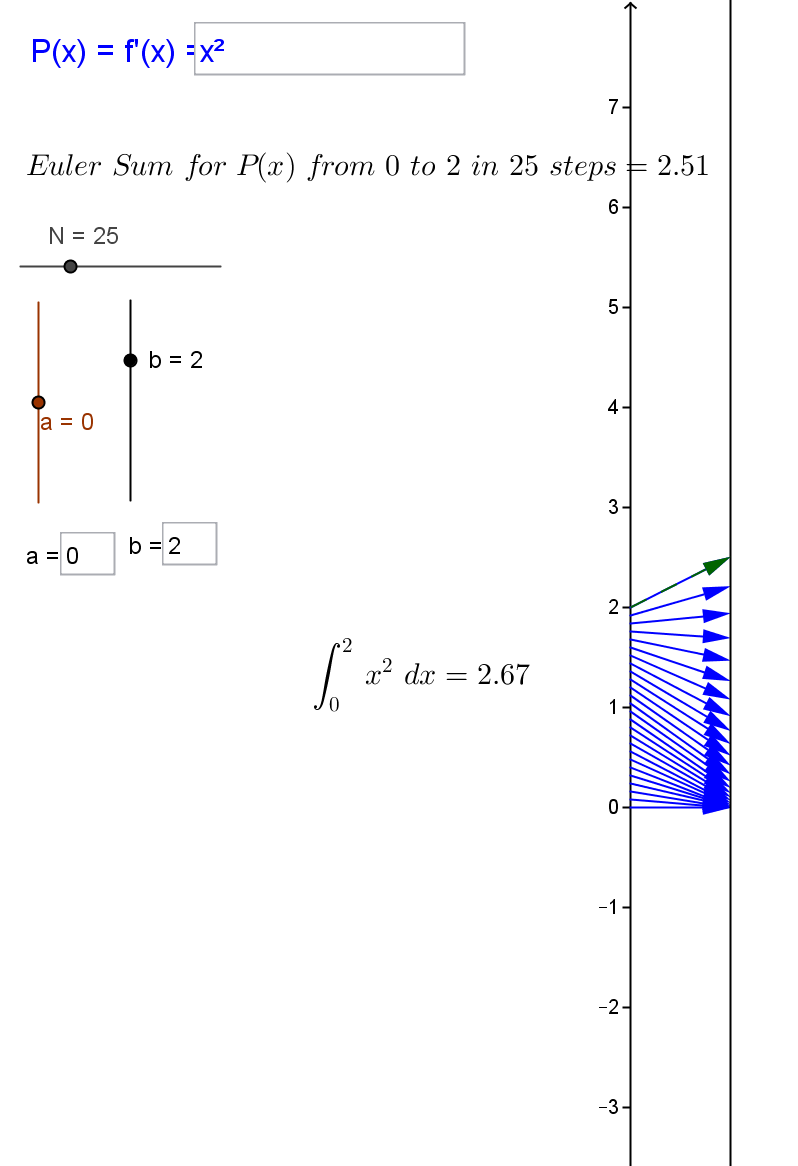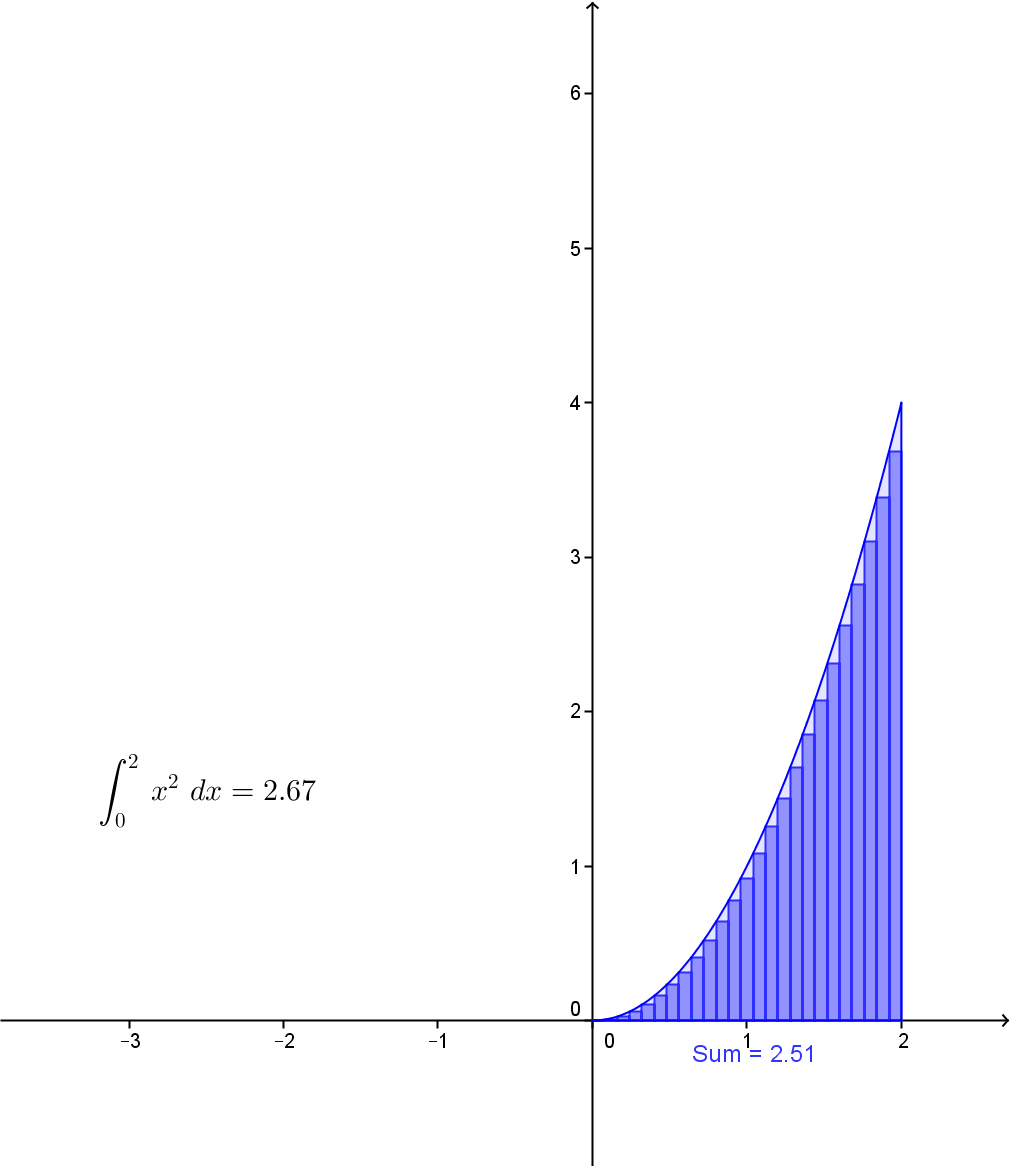This is a Java Applet created using GeoGebra from www.geogebra.org - it looks like you don't have Java installed, please go to www.java.com
Theorem: Suppose $P(x)$ is a continuous function on $[a,b]$, then $\int_a^b P(x)dx$ exists. ¤

5.2  The Additive Property for Integral
Theorem:
[The Additive Property.]  Suppose $y = P(x)$ is a continuous function on $[a,b]$ and $a<c<b$ then
$$\int_a^c P(x)dx + \int_c^b P(x)dx = \int_a^b P(x)dx$$
In General: $\int_a^b P(x) dx = \int_a^c P(x) dx + \int_c^b P(x) dx$ for any $a, b,$ and $c$.

Motion Interpretation: Assume  $a < c < b$. The net change in position of an object moving with velocity $P$ between time $a$ and time $b$, $\int_a^b P(x) dx$, is the sum of the net changes in position between time $a$ and time $c$, $\int_a^c P(x) dx$, and between time $c$ and time $b$, $\int_c^b P(x) dx$. See Figure V.B.1.
Geometry Interpretation: If $P(x) > 0$ and $a < c < b$ , then the area of the regions above the $X$-axis and below the graph of $Y=P(X)$ between the lines $X=a$ and $X=b$, $\int_a^b P(x) dx$ , is the sum of the areas of the regions enclosed by the $X$-axis, the graph of $Y=P(X), X=a$ and $X=c$, $\int_a^c P(x) dx$ , and by the $X$-axis, the graph of $Y=P(X), X=c$ and $X=b$,  $\int_c^b P(x) dx$  .
See Figure V.B.1.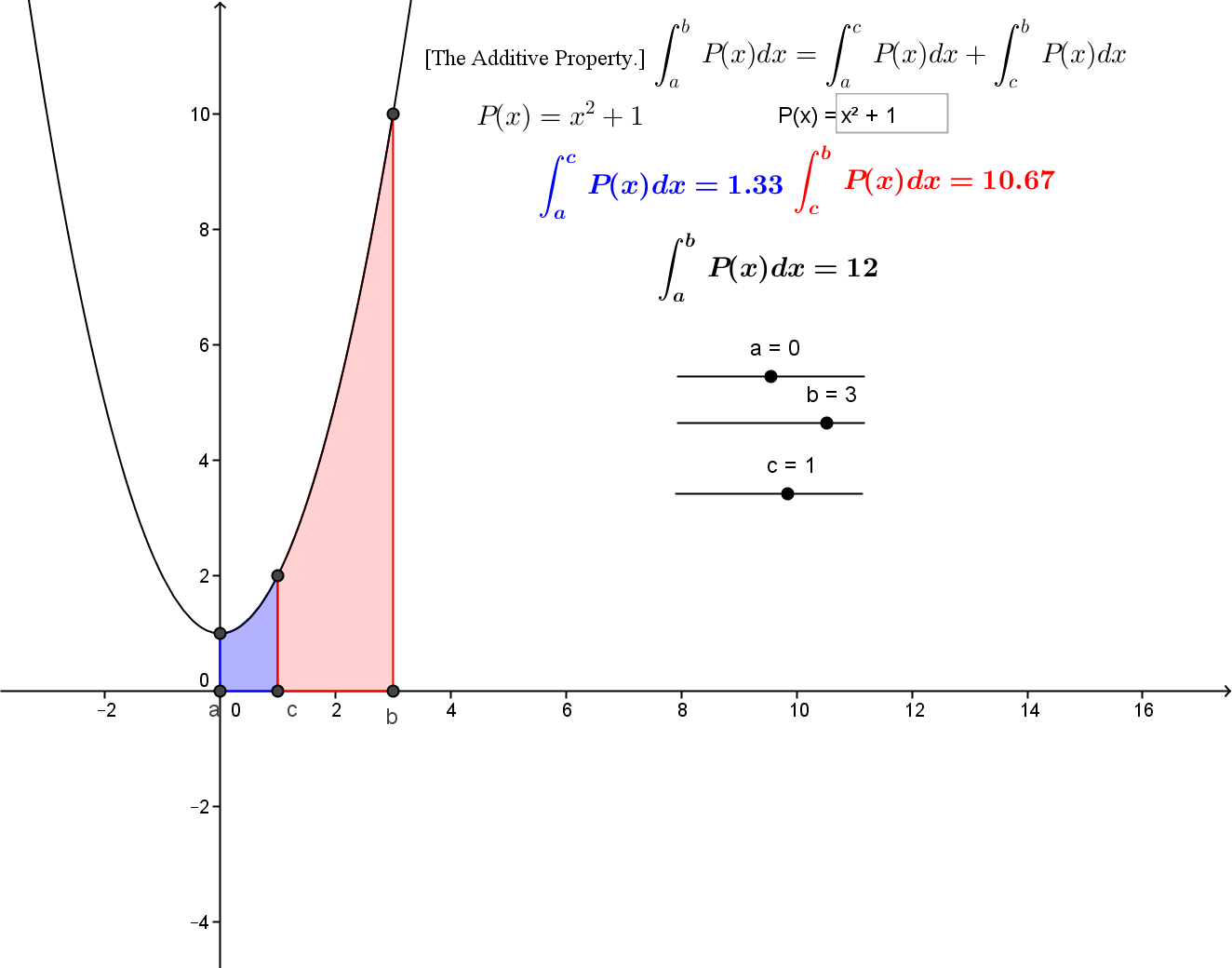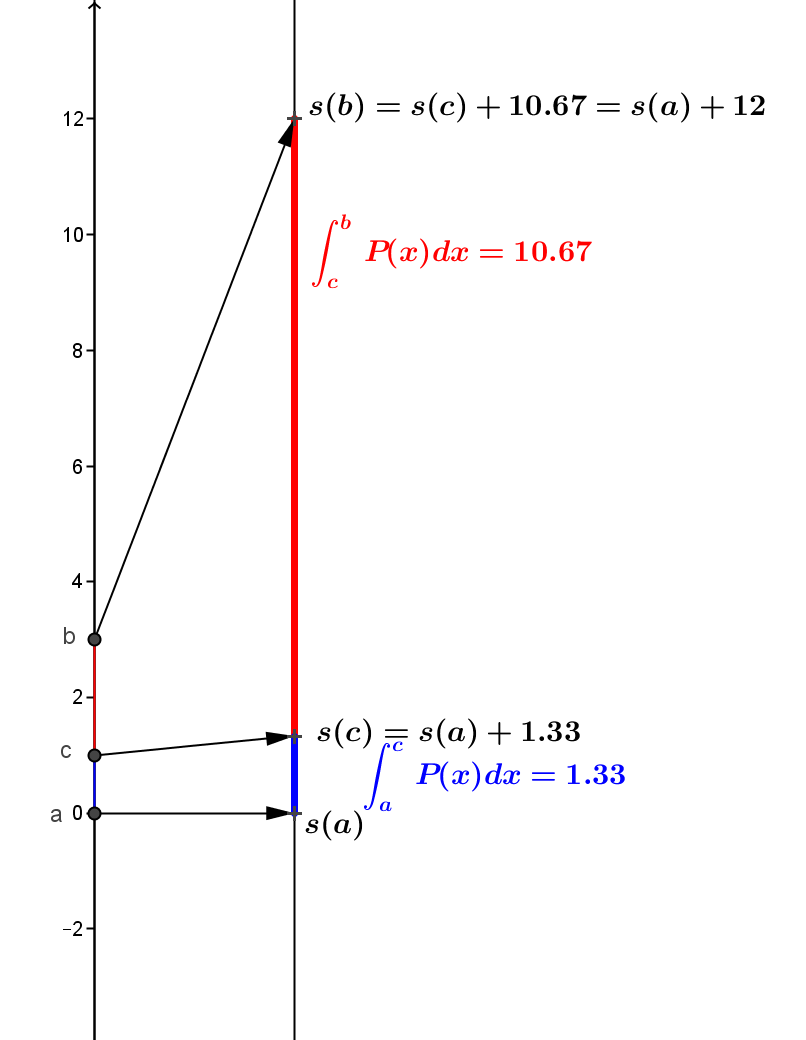Figure V.B.1. This is a Java Applet created using GeoGebra from www.geogebra.org - it looks like you don't have Java installed, please go to www.java.com
Move the sliders to change $a,b$, and $c$. You can also change the function $P(x) = f'(x)$ by entering a new function in the box.¤

The additive property of the definite integral is visualized with mapping diagram and graph below, showing the connection between the integral estimates with the mapping diagrams and the areas of regions in the plane bounded by the graph of
$y = P(x) = f'(x)$, the X axis, X=a, X = b, and X =c.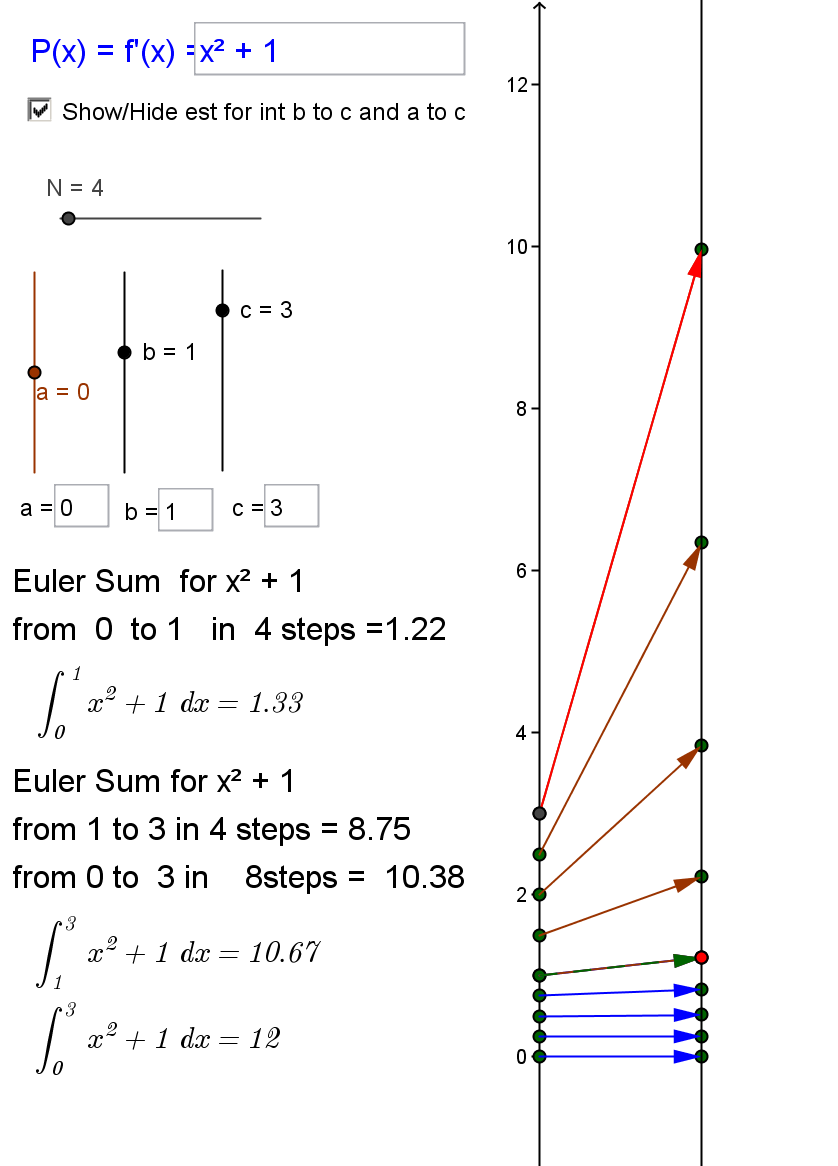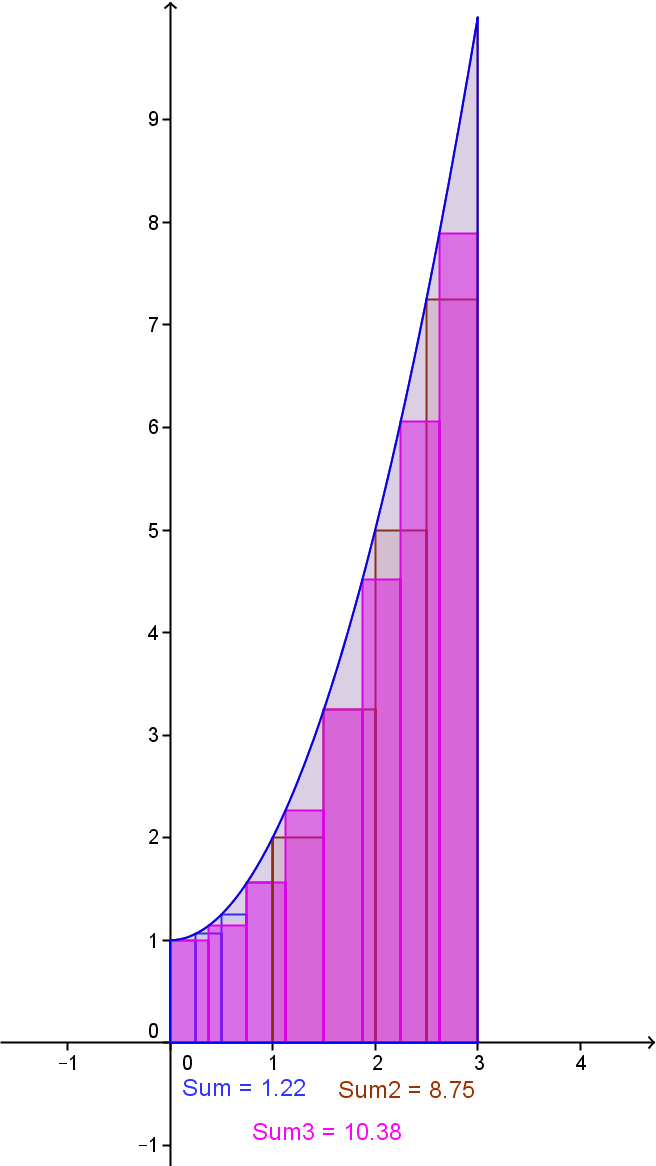This is a Java Applet created using GeoGebra from www.geogebra.org - it looks like you don't have Java installed, please go to www.java.com
Move the sliders to change $a,b,c$, and $N$. You can also change the function $P(x)$ by entering a new function in the box.

5.3 Mean Value Theorem for Integrals:¤
If $P$ is a continuous function on $[a,b]$ then there is an number $c_*$ between $a$ and $b$ where
$$\int _a^b P(x) dx = P(c_*)*(b-a).$$

This is a Java Applet created using GeoGebra from www.geogebra.org - it looks like you don't have Java installed, please go to www.java.com

5.4 The Fundamental Theorem of Calculus.
¤  Suppose $y = P(x) = f'(x)$ is a continuous function, then
$$\int_a^b P(x)dx + f(a) = f(b)$$
or
$$\int_a^b P(x)dx = f(b) - f(a)$$
where $f'(x) = P(x)$.

5.4.1 Euler's Method visualized with mapping diagram and graph, showing the connection for the Fundamental Theorem of Calculus between the mapping diagram and estimating
$$\int_a^b P(x)dx = f(b) - f(a)$$ where $f'(x) = P(x)$
 x f(x) f'(x) df=f'(x)dx 0. 0 1.0 0.2 0.2 0.2 1.4 0.28 0.4 0.48 1.8 0.36 0.6 0.84 2.2 0.44 0.8 1.28 2.6 0.52 1.0 1.80 3.0 0.60 1.2 2.40 3.4 0.68 1.4 3.08 3.8 0.76 1.6 3.84 4.2 0.84 1.8 4.68 4.6 0.92 2.0 5.60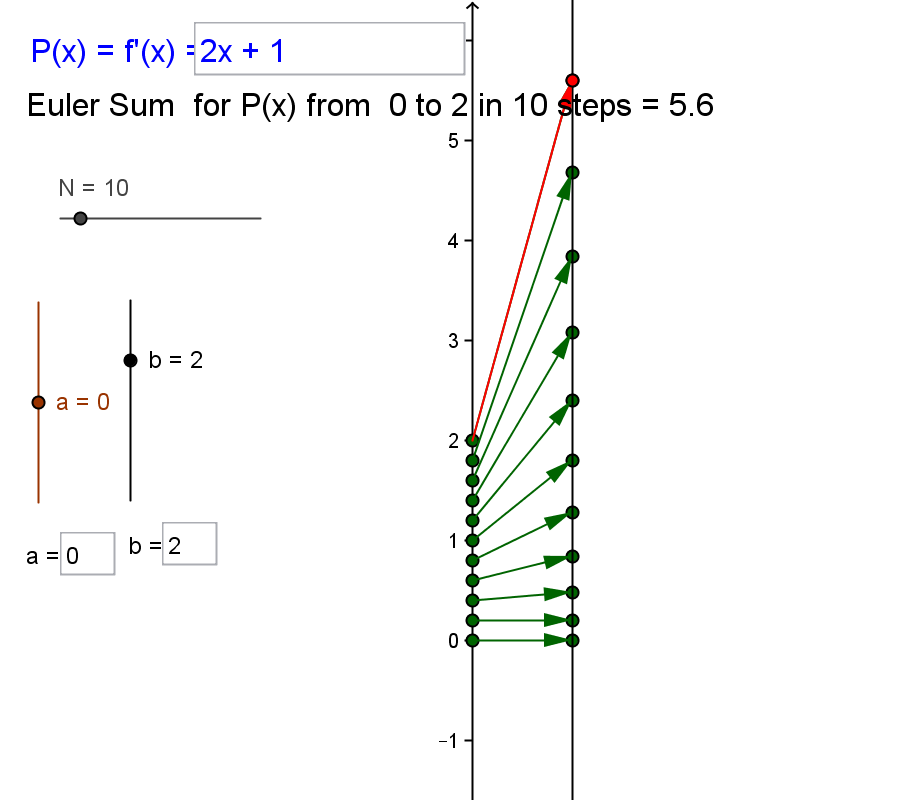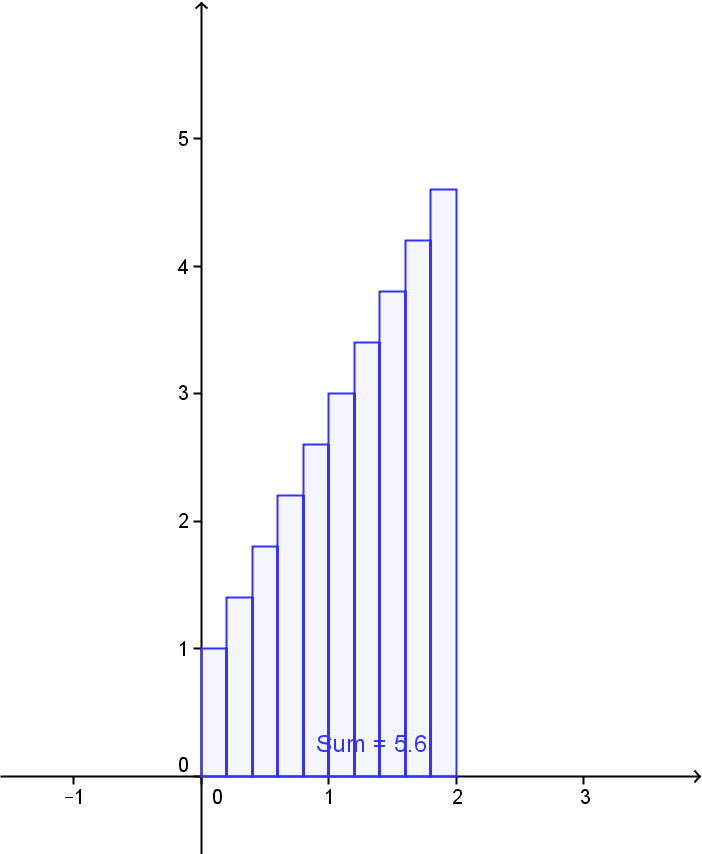This is a Java Applet created using GeoGebra from www.geogebra.org - it looks like you don't have Java installed, please go to www.java.com

Move the sliders to change $a,b$, and $N$. You can also change the function $P(x) = f'(x)$ by entering a new function in the box.

In context of the Sensible Calculus Text: IV.F Euler's Method Meets The Position, Cost, and Area Problem

5.4.2 Euler's Method visualized with mapping diagram and graph, showing the connection for the Fundamental Theorem of Calculus between the mapping diagram and
$$\int_a^b P(x)dx$$ where $f'(x) = P(x)$ evaluated by GeoGebra's CAS. ¤

This is a Java Applet created using GeoGebra from www.geogebra.org - it looks like you don't have Java installed, please go to www.java.com
Move the sliders to change $a,b$, and $N$. You can also change the function $P(x) = f'(x)$ by entering a new function in the box.

6 & 7.Sequences and Series

6.1 GeoGebra Applet for visualizing  sequences with Mapping Diagrams and Graphs for a sequence defined by $a_n = f(n)$. Examples.¤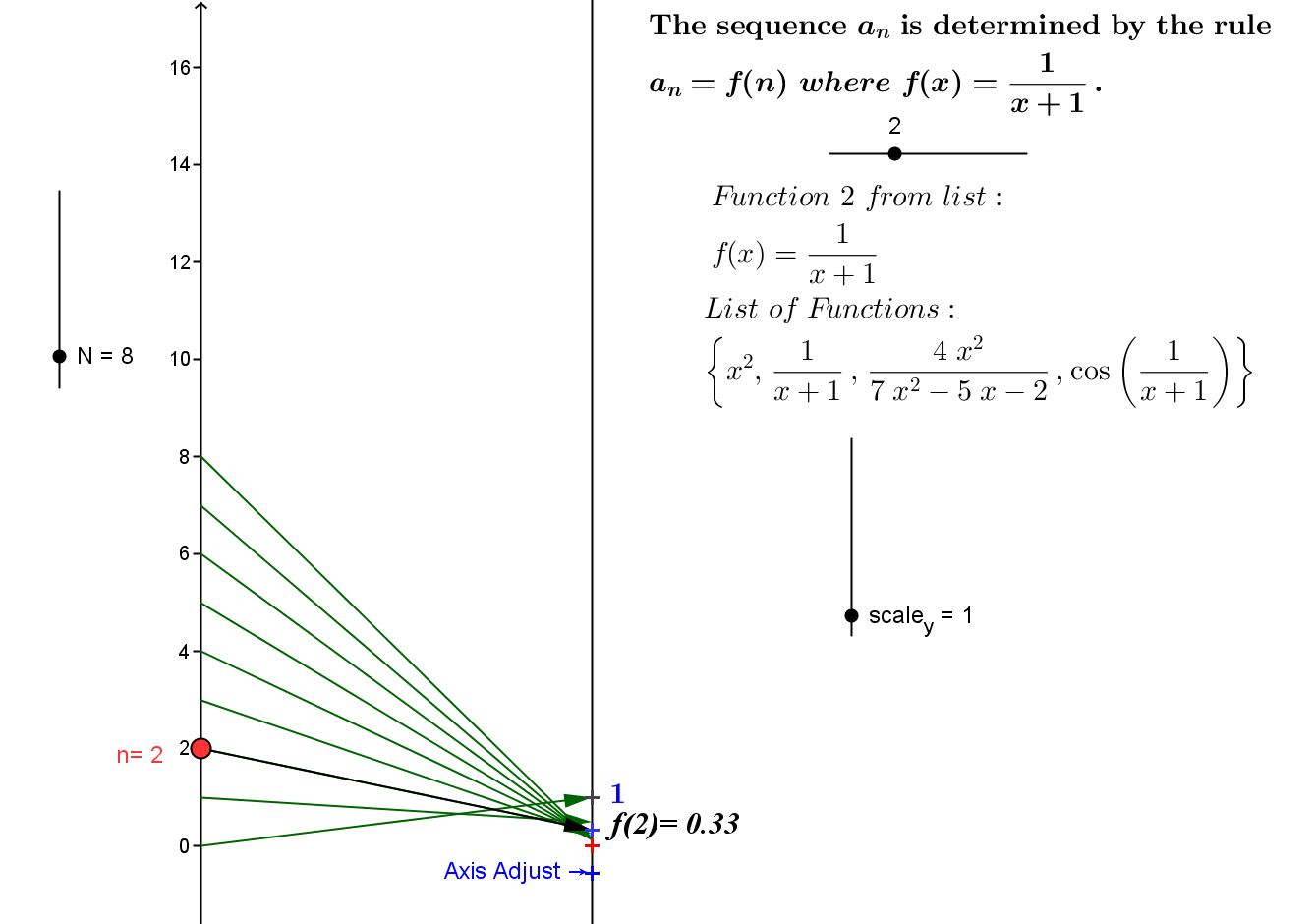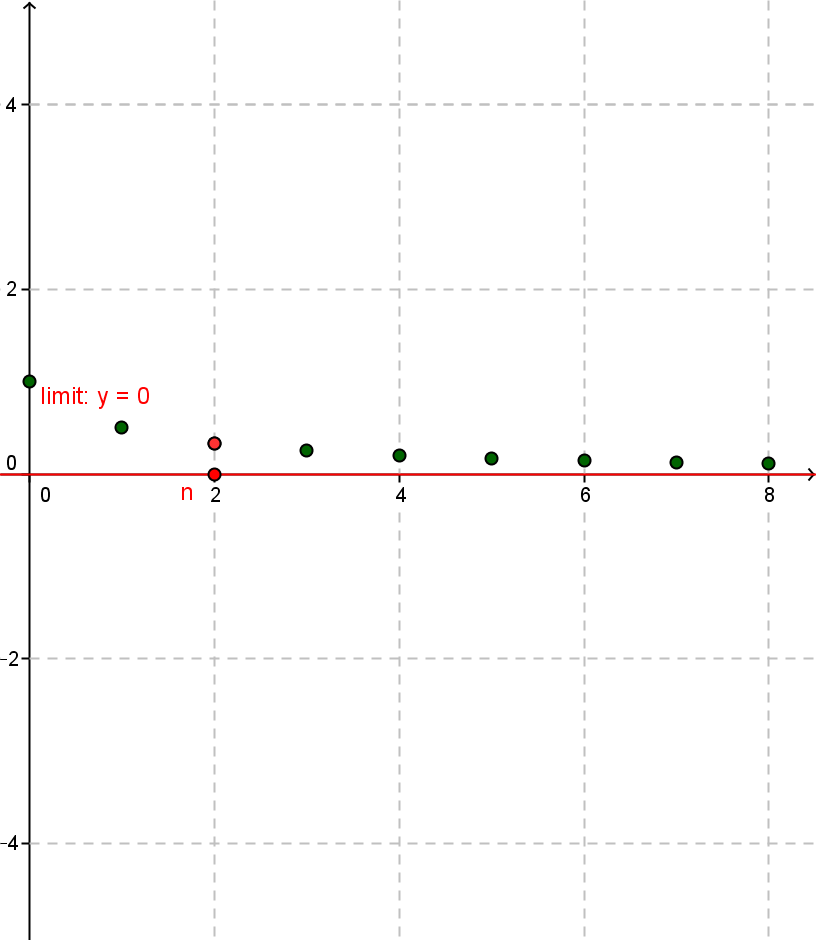This is a Java Applet created using GeoGebra from www.geogebra.org - it looks like you don't have Java installed, please go to www.java.com

6.2 GeoGebra Applet for visualizing  sequences with Mapping Diagrams and Graphs for a sequence defined by $a_n = f(n)$.¤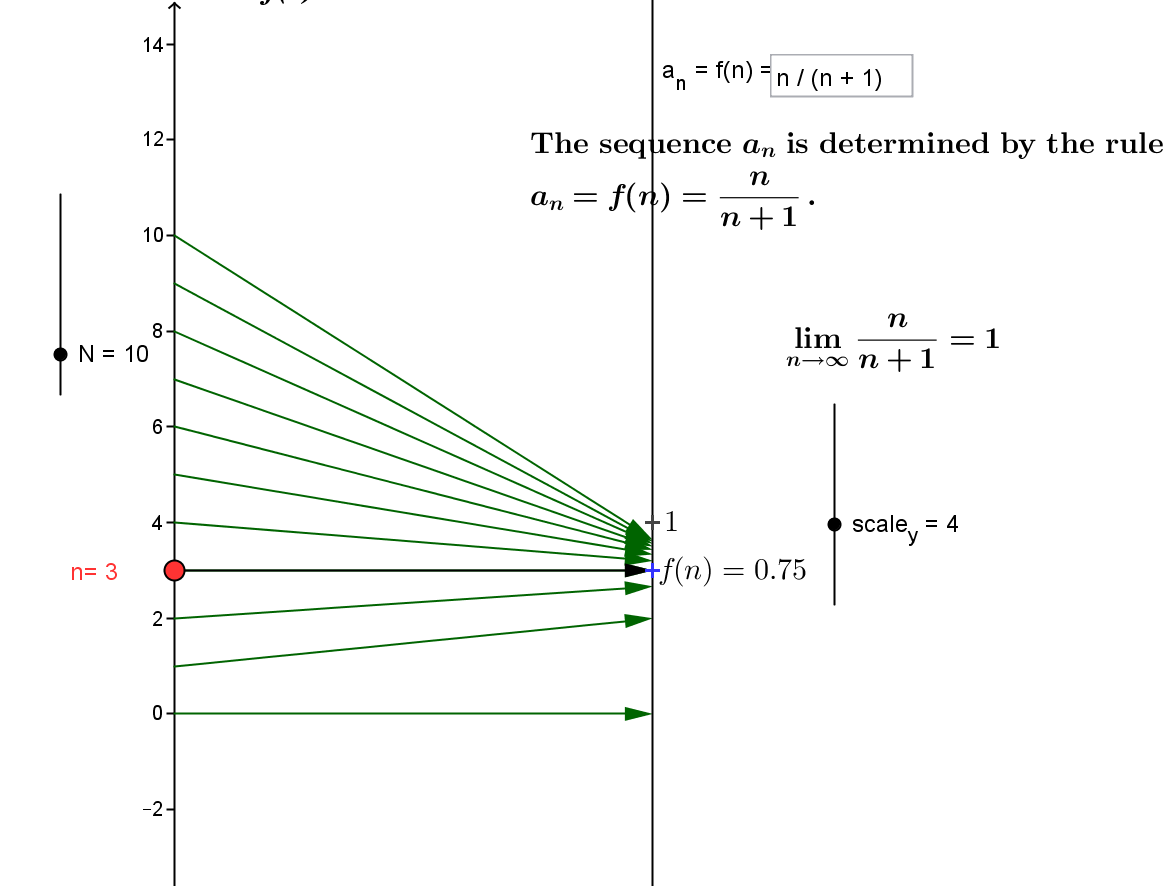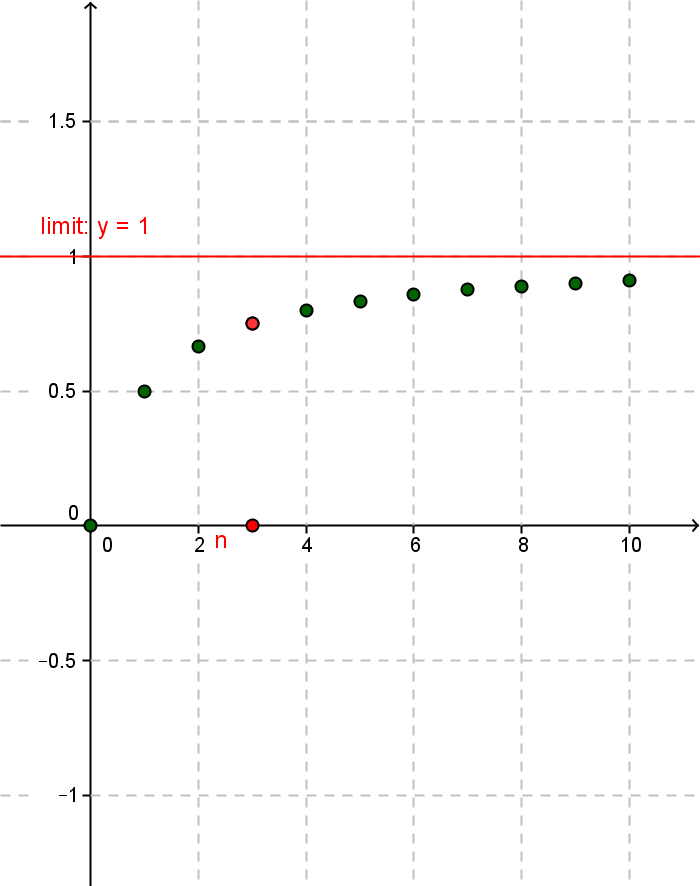This is a Java Applet created using GeoGebra from www.geogebra.org - it looks like you don't have Java installed, please go to www.java.com

7.1 GeoGebra Applet for constructing MacLaurin Polynomials and Remainders $P_n(x,f(x))$ and $R_n(x,f(x))$¤

This is a Java Applet created using GeoGebra from www.geogebra.org - it looks like you don't have Java installed, please go to www.java.com

8. Multi-dimensional Calculus

Visualizing $z=f(x,y)$ and Understanding Partial Derivatives and the Differential in 3D with GeoGebra 5.0
A glimpse of multi-dimensional calculus with mapping diagrams.
Mapping Diagrams add much to visualizing multi-variable functions.

8.1 : Linear Functions of 2 variables: $z =f(x,y) = Ax + By + C$. As with functions of one variable, understanding linear (affine) functions is a key to the calculus of multi-variable functions, Mapping diagrams provide a valuable tool to understanding visually linear functions with 2 or more variables.¤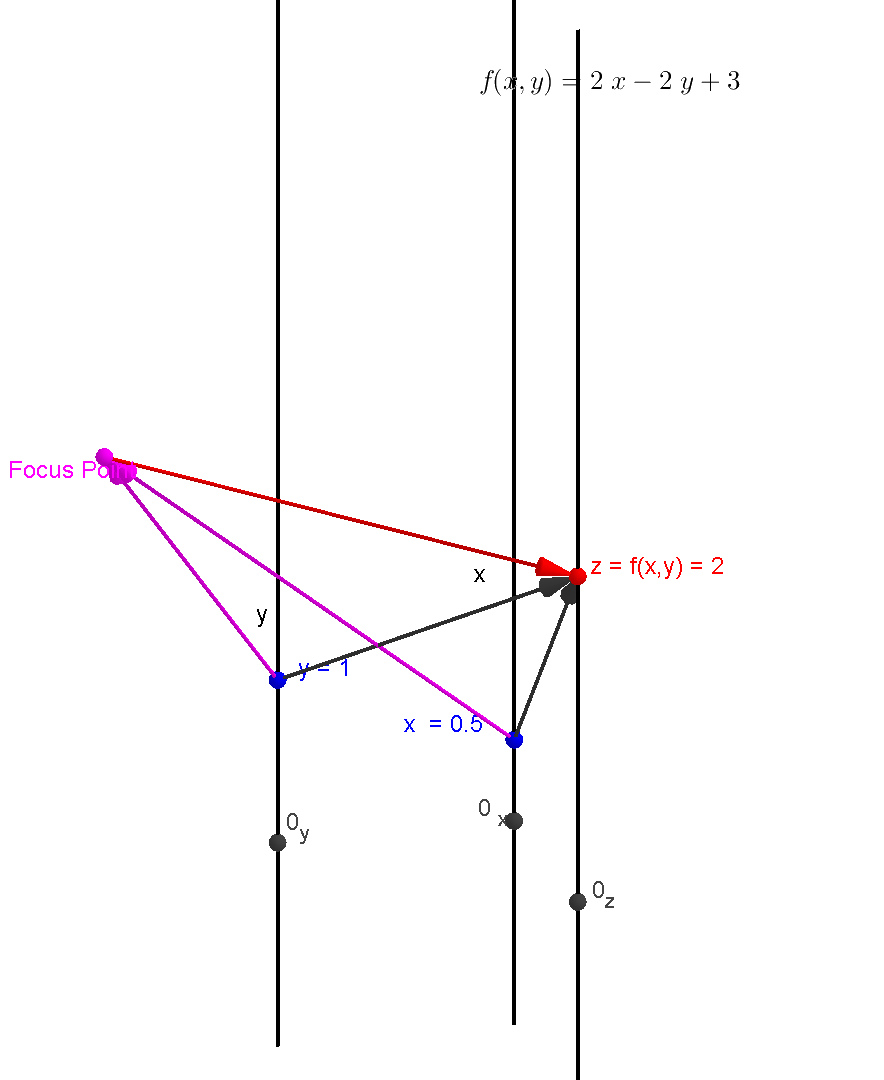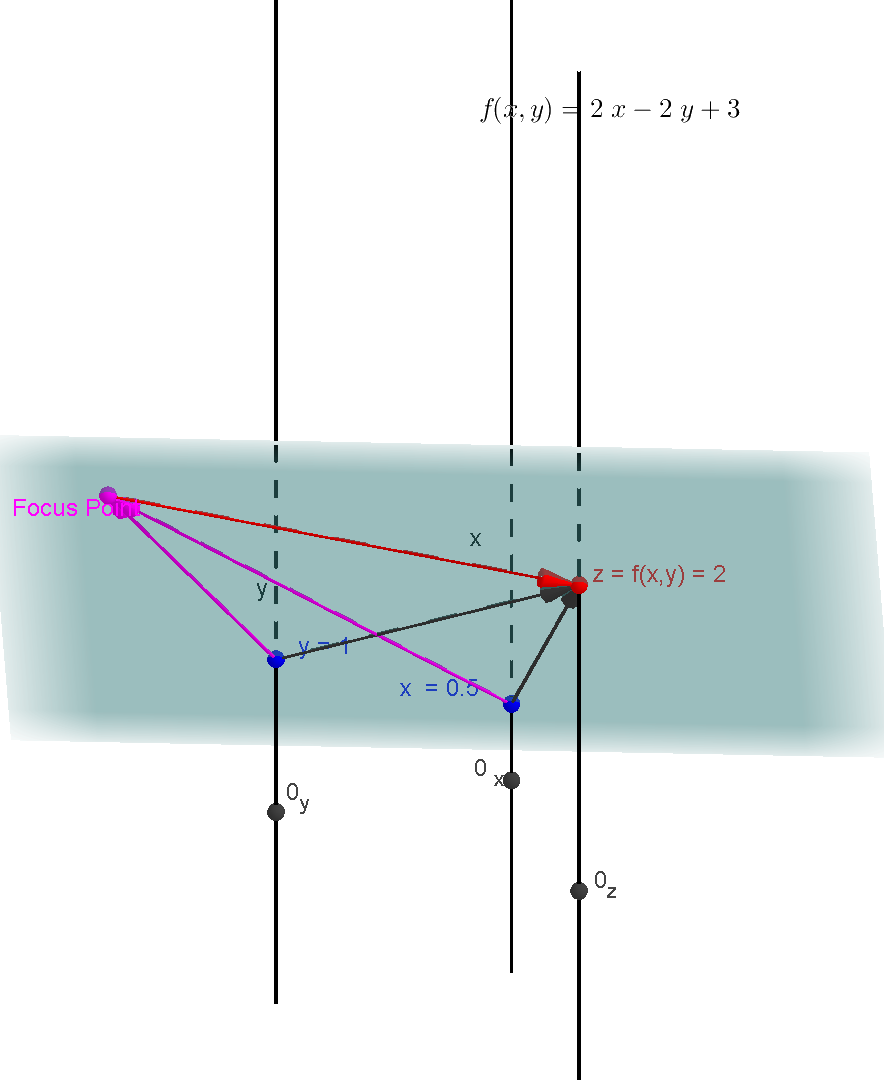A mapping diagram for a linear function of 2 variables exhibits a focus point in a 3-D image.
This point completely determines the linear function values.
Using the plane determined in space by the three points: x on the x - axis, y on the y-axis, and the focus point,
the value of the function is determined by where that  plane intersects the z axis.

8.2:  Functions of 2 variables: $z =f(x,y)$ and partial derivatives. As with functions of one variable, functions of many variables are understood with linear functions (a key to the calculus of multi-variable functions), partial derivatives and the differential. Mapping diagrams provide a valuable tool to understanding visually these concepts for functions with 2 or more variables.¤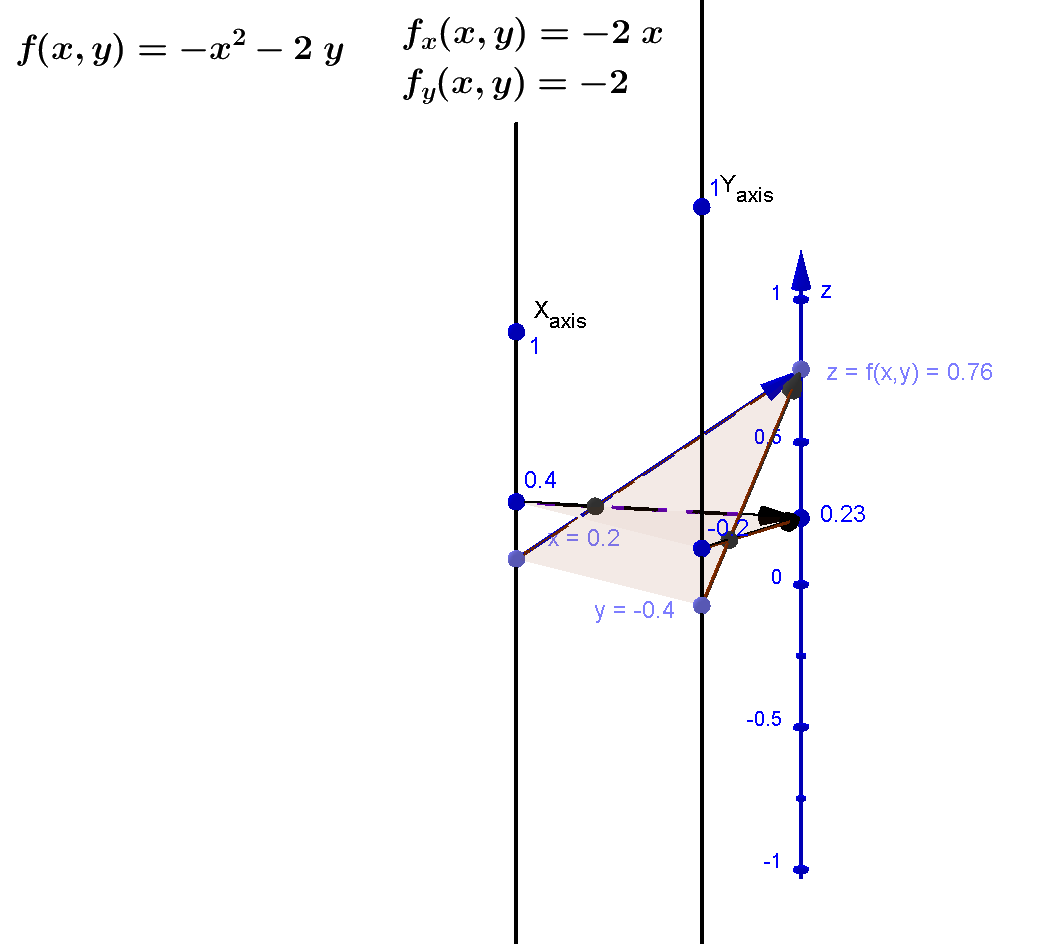Mapping diagram for $f(x,y) = -x^2 -2y$ with evaluation for two pairs of data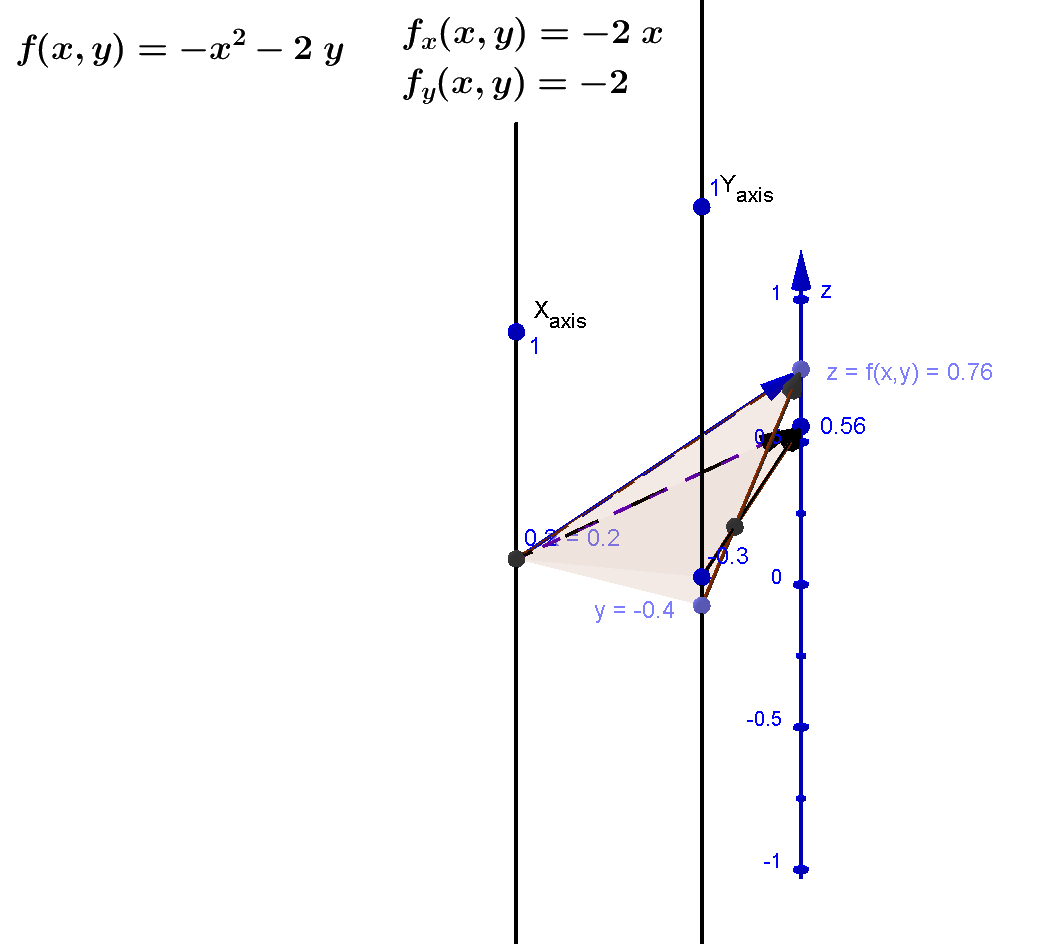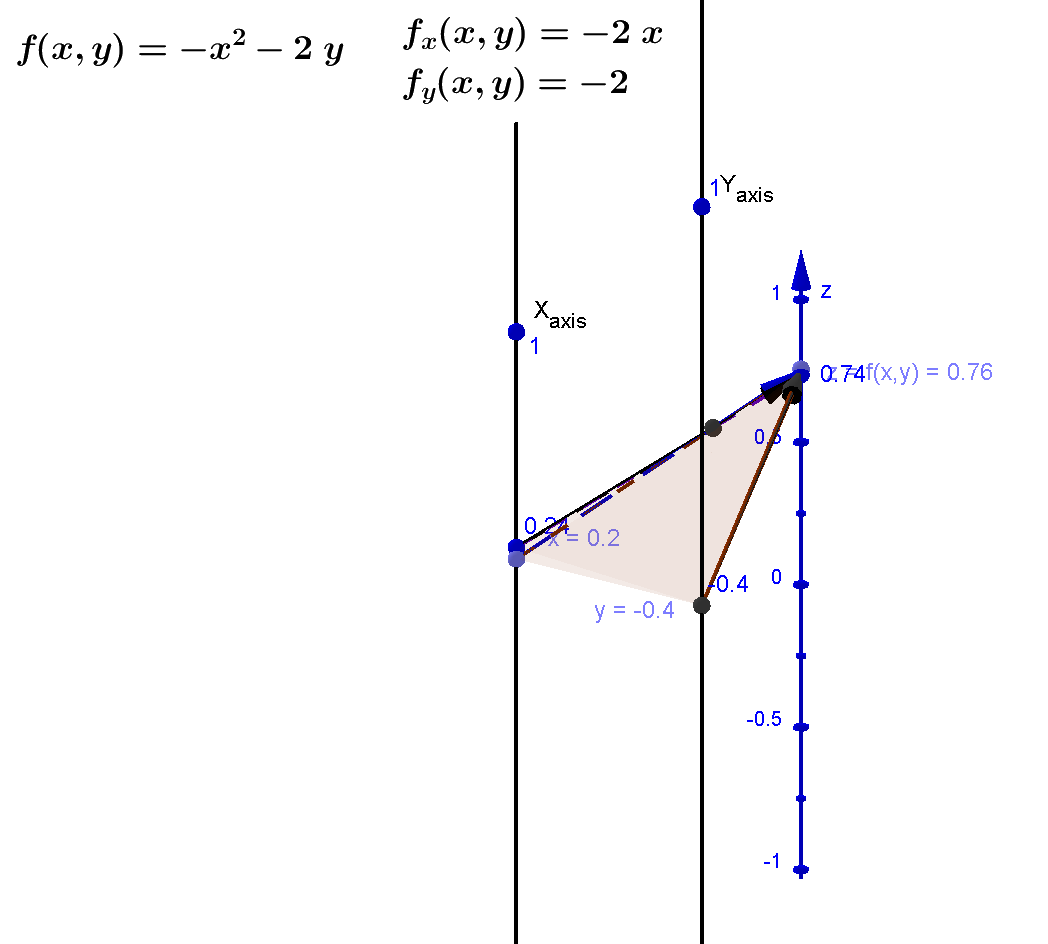Mapping diagram for $f(x,y) = -x^2 -2y$
illustrating estimates for partial derivatives $f_x(0.2,-0.4)$ and $f_y( 0.2,-0.4)$.

Thanks for participating
¤.

Questions?

The End!